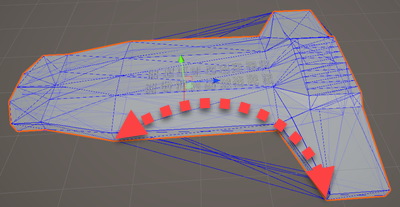# [SOLVED] What is wrong with my Mesh.Triangle code? [wrong iteration pattern]

Its probably obvious, but I can’t seem to get the logic to get the correct triangle shapes/placements and basically draw a wire mesh overlay.

I’m going through a list of triangles in my code, and drawing a blue line for each of the edges.

``````        Gizmos.color = Color.blue;
var v = meshFilter.mesh.vertices;

var draw = new System.Action<Vector3, Vector3>((a, b) =>
{
Gizmos.DrawLine(
transform.TransformPoint(a),
transform.TransformPoint(b));
});

foreach (int t in meshFilter.mesh.triangles)
{
if (t + 3 > v.Length) break;

draw(v[t], v[t + 1]);
draw(v[t], v[t + 2]);
draw(v[t+2], v[t + 1]);
}
``````

The first weird part is that in my model, the highest index returned from mesh.Triangles is 325, but the highest vertex index is 326. My understanding is that triangle returns the first index of 3 vertices, meaning I expected vertices 325, 326 & 327. 327 is out of bounds of the vertex array.

The second weird part is the drawn lines for the remaining parts:While the triangles in general are correctly placed, there are clearly extra lines being drawn where there are no faces. I checked in Blender, and there are no edges not connected to a face.

It seems I’m grabbing the wrong vertices for the triangles, but I’m not sure why. Any ideas?

Edit (preface): The triangle (int) data held in a Mesh class is meant to be used in sets of three, where each set corresponds to the three vertex indices in the vertex (Vector3) data.

You're using a foreach() loop incorrectly. Use a for() loop for this.

I’ll describe a simple example: Let’s say the first two triangle index sets are…

``````(0, 1, 15)
(14, 32, 17)
``````

… So, in other words, the first two triangles would use those described vertex indices.

Here’s what your foreach() loop does right now when converted into a for() loop:

``````for(int t = 0; t < meshFilter.mesh.triangles.Length; t++)
{
draw(v[triangles[t]], v[triangles[t] + 1]);
draw(v[triangles[t]], v[triangles[t] + 2]);
draw(v[triangles[t] + 2], v[triangles[t] + 1]);
}
``````

… when compared with the two example triangles, here’s the converted output:

``````// t = 0
draw(v, v[0+1]);
draw(v, v[0+2]);
draw(v[0+2], v[0+1]);
// t = 1
draw(v, v[1+1]);
draw(v, v[1+2]);
draw(v[1+2], v[1+1]);
// t = 2
draw(v, v[15+1]);
draw(v, v[15+2]);
draw(v[15+2], v[15+1]);
// t = 3
draw(v, v[14+1]);
draw(v, v[14+2]);
draw(v[14+2], v[14+1]);
// t = 4
draw(v, v[32+1]);
draw(v, v[32+2]);
draw(v[32+2], v[32+1]);
// t = 5
draw(v, v[17+1]);
draw(v, v[17+2]);
draw(v[17+2], v[17+1]);
``````

By this example, vertices 2, 3, 16, 18, 19, 33, and 34 are all being used to draw 6 triangles where only two are defined.

Your for() loop should look more like this:

``````Mesh mesh = meshFilter.mesh;
List<int> triangles = new List<int>();
mesh.GetTriangles(triangles, 0);
for(int i = 0; i < triangles.Count; i+=3)
{
// including zeroes for simplified spacing example
draw(v[triangles[i + 0]], v[triangles[i + 1]]);
draw(v[triangles[i + 1]], v[triangles[i + 2]]);
draw(v[triangles[i + 2]], v[triangles[i + 0]]);
}
``````

Facepalm… I was iterating in steps of 3 in exactly the wrong spot… Thanks!